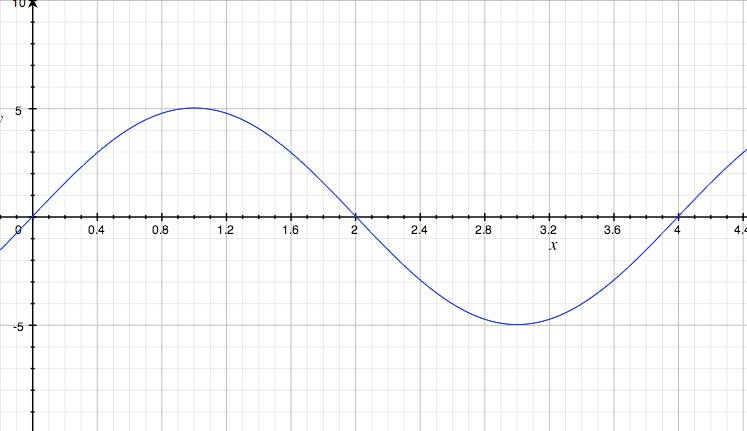SEARCH HOMEMath Central Quandaries & QueriesKim, a student: I am given a graph with a wave. The amplitude is 5cm and the period is 4cm. I am suppose to find the angular speed. What I need to know is the formula for angular speed and how do I use these numbers to get the correct answer. Thanks KimHi Kim,

I assume your graph looks like the following:I assume you mean that the period is 4 seconds. The formula for angular speed is ω=2 π/T where ω is angular speed and T is period length. The unit for angular speed is radians/second. If you want to find the speed in cm/second, that is actually linear speed. The angluar - linear speed relation is v=r*ω where v is linear velocity and r is radius. Since you are given a sine graph showing distance versus time, your amplitude shows what the radius is for your angular speed.

Hope this helps,

JaniceMath Central is supported by the University of Regina and The Pacific Institute for the Mathematical Sciences.